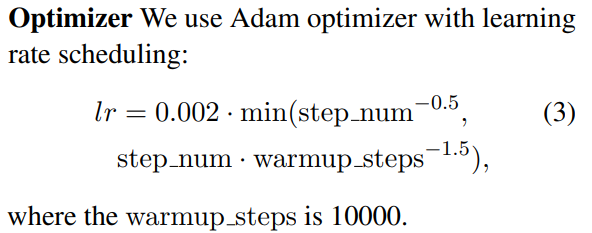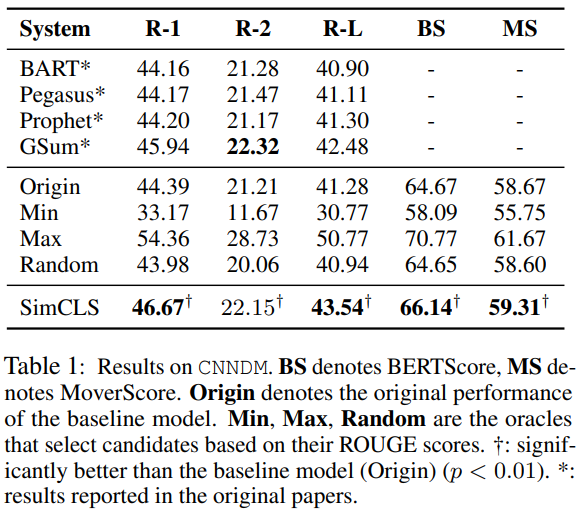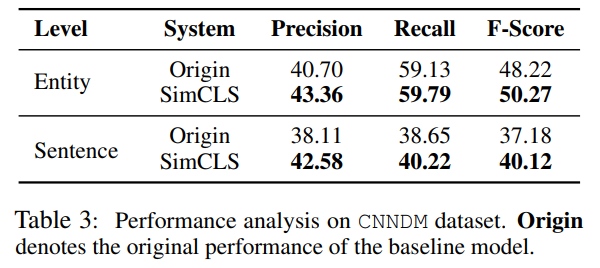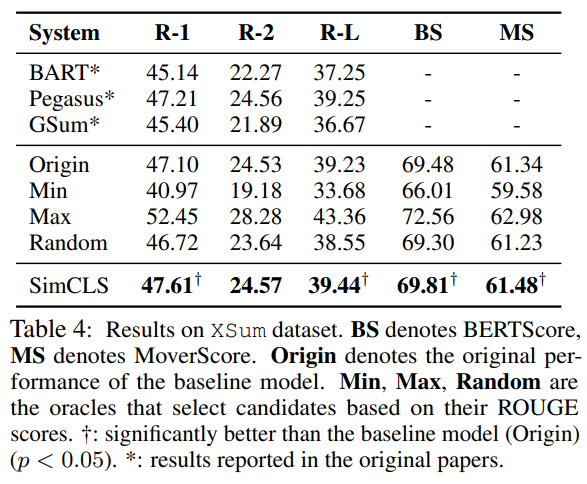《SimCLS: A Simple Framework for Contrastive Learning of Abstractive Summarization》（SimCLS，ACL 2021）

yixinL7/SimCLS (github.com)

## 摘要

• 将文本生成形式为无参考评估问题+对比学习
• 在CNN/DailyMail数据集上，比BART提高2.51，比PEGASUS提高2.50

## 介绍

• seq2seq通常在最大化似然估计（MLE）的框架下使用，trained with teacher-forcing——使得目标函数和评估方法存在gap，目标函数基于token-level的预测，评估方法会对整体的语句进行评估，并且自回归生成会带来错误积累
• 上述的训练与测试之间的差异称为exposure bias，本文使用对比学习，实现了用相应的评估指标直接优化模型的objective，从而减小了上述的差异
• 部分文章（Contrastive learning with adversarial perturbations for conditional text generation，2021 ICLRContrastive learning for many-to-many multilingual neural machine translation）已经尝试在条件文本生成里将对比损失引入到MLE训练，本文在框架的不同阶段引入对比损失和MLE损失来分开二者的作用
• 本文为two-stage：
• 首先训练一个seq2seq，生成候选摘要（由MLE损失训练）
• 其次训练一个评估模型（通过对比学习训练），为候选摘要打分

## 抽象摘要的对比学习框架

• 给定一个文本$$D$$和摘要$$\hat{S}$$，抽象摘要模型$$f$$需要生成候选摘要$$S=f(D)$$，使得分数$$m=M(S,\hat{S})$$最大

• 本文将整个生成过程分为两个阶段，包含生成模型$$g$$和评估模型$$h$$，后者用于打分和选择最佳候选摘要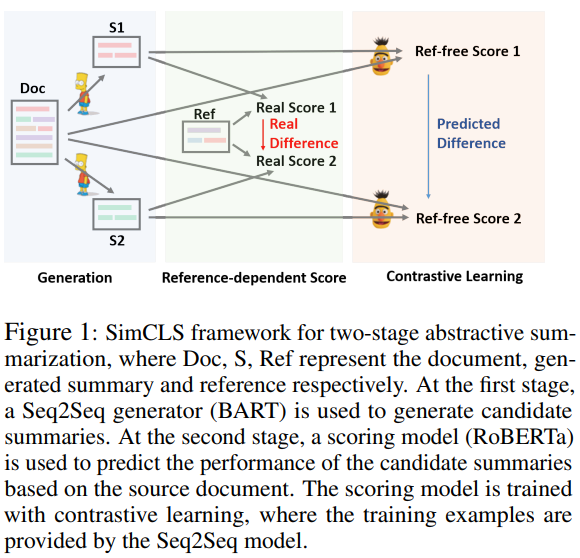• 阶段I：候选生成

• $$g$$为一个预训练的seq2seq模型，最大化MLE
• 由Beam Search生成候选摘要$$S_1,...,S_n$$
• 阶段II：无参考评估

• $$h$$为一个预训练模型（本文使用Roberta），用于给生成的候选打分：$$r_i=h(S_i,D)$$，并且最终输出摘要$$S=argmax_{S_i}h(S_i,D)$$
• $$h$$分别编码$$S_i$$$$D$$，第一个token（即CLS）之间的cosine similarity即为相应的相似得分$$r_i$$
• 对比学习

• 这里的“对比性”反映在$$h$$评估的生成摘要的不同质量中

• $$h$$引入排名损失：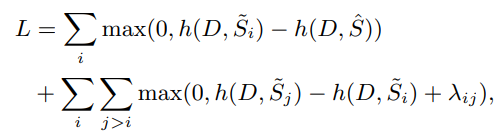• 其中$$\widetilde S_i$$为根据$$M(\widetilde S_i,\hat S)$$的降序排序结果
• 其中$$\lambda_{ij}=(j-i)*\lambda$$$$\lambda$$为超参数，设置为0.01
• $$M$$为评估方法，例如ROUGE

## 实验

• 使用CNN/DailyMail、XSum

• 使用ROUGE-1/2L作为主要的评估方法

• 生成模型和评估模型分别训练：使用BART和Pegasus作为生成模型，Roberta作为评估模型

• 使用Transformers提供的checkpoint，并使用diverse beam search作为采样方案（16 groups）